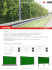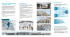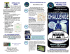# BRIDGE OPTIMIZATION FOR THERMISTOR DC = d~T = ~T (I) (2)

## Transcription

BRIDGE OPTIMIZATION FOR THERMISTOR DC = d~T = ~T (I) (2)
```J ournal of Glaciology, Vol. 16, No. 74, 1976
BRIDGE OPTIMIZATION FOR THERMISTOR
MEASUREMENTS
By B.
BARRY NAROD
(Department of Geophysics and Astronomy, University of British Columbia, 2075 W esbrook
Place, Vancouver, British Columbia V6T IW5, Canada)
ABSTRACT. An expressio n for optimum bridge parameters is derived for a thermistor in a Wheatstone
bridge a nd numeri ca l va lues a re assig ned to d e termine the useful limits of resistance bridges for thermistor
measurements. Several digita l ohmmeters a r e evalua ted as m eas uring devices a nd a r e shown to compare
unfavo urably wi th a simpl e bridge a nd null detector.
RESUME. Oplimiza lion d 'ull pOlll de mesures a lhermislor. On e tablit un e expression pour les parametres d ' un
pont optimum pour un thermistor inclu d a ns un pont d e Whea tsto ne et I'on donne d es va leurs nume riques
pour d eterminer les limites utilisabl es des ponts a resistance p o ur d es mesures au thermistor. Plusie urs
ohm etres digitaux sont juges en ta nt que dispositif de mesure et I'o n montre que la comparaison ne leur es t
pas favorable avec un simpl e pont a m esure de zero.
ZUSAMM ENFASSUNG. Oplimierllllg der W iderslandsbriicke Jilr Thermislor-M essungelZ. Fur einen Th erm istor in
ein er Wh eatstonesch e n Brucke wi rd ein Ausdruck fur optima le Bruckenparameter he rgeleite t. Zur Bestimmun g d er zweckmassigen Be m essung vo n Wid e rstanclsbrucken fur Th e rmistor-Mess unge n werden Za hl e nwerte
angegeben. AIs Me3Svorri chtung werd en versch iedene digitale Ohmmeter untersu cht, wobei sich zeigt,
dass sie im Vergleich mit e iner einfachen Bruc ke unci einem N ull-De tektor ungunstig a bschn eiden.
INTROD UCTION
In general, thermistor s, when used as temperature m easuring devices, are used singly or
in pairs in some impedan ce net, that n et being driven by som e power supply, and also hav ing
an output which is m onitored by a d etector. Frequently the thermistor is placed at th e end
of a transmission line.
Errors in precision and accuracy enter from nume rous sources: from thermistor drift,
from the power suppl y o r its configuration , from the d e tector, impedance n e t or transmission
line, and from the calibration fit (Beck, 1956; Misen er a nd T hompson , 1952 ; Muller and
Stolton, 1953; Fen wal, I 968 [aJ). It is the intent of this paper to examine instrum ental
parameters, a nd to discu ss how they m ay be used or d etermin ed , in ord er to optimize the
precision with which th e r esistance ofa given thermistor in a fixed situation can be determined.
These para meters include: thermistor self-h eating, nominal thermistor r es istance, range of
appli cation, and resolution of the d etector. This examination applies equall y both to the
calibration of the sen sor and to field m easurements u sing it since both conditions can be
completely described.
THERMISTOR CH AR ACTE RISTICS
Manufacturers of thermistors generally provide figures for their thermistors that indicate
the abi li ty of the d evice to dissipate power P into the surrounding medium . This " dissipation
constant" Dc is specifically d efin ed as th e amount of powe r required to raise the temperature
of the thermistor I deg above the ambient temperature T. More conveni ently
dP
P
DC = d~T = ~T
(I)
where t1 T is th e magnitude of thermistor self-heating. In ice, for spherical thermistors
(Carslaw and Jaeger , 1959),
D c = 47Tk i rt
(2)
where ki is the thermal conductivi ty of ice and Tt is th e radius of the thermistor. Equation ( 1)
clearly d epends on the environm ent of the thermistor. Manufacturers ' figures are usually
26 9
27 0
JOURNAL
OF
GLAC10LOGY
given for the thermistor in still air. In ice D c is at least a factor of three greater, and although
increases in D c will improve the measurem ent precision, a factor of three in D c results in no
more than 1.5 dB improvement in precision . H ence, for th e examples u sed h ere, manufacturers' figures will be used.
Thermistor have negative thermal coe ffi cients of resistivity, which shall b e call ed RT.
For most thermistors
and this figure will be used in all of the examples (J essop and Judge, 1974) .
The " tim e constant" of a thermistor, as d efin ed by some manufacturers is the time required
for a thermistor to change its temperature 63 % of the amount of temperature change of a
value impressed upon it in a step change (Fenwal, 1968[b] ) . For a given thermistor, this may
vary from fractions of seconds to minutes as a function of environment. For steady-state
temperature m easurements, a more useful time constant would be the time required for a
thermistor to reach 63 % of 6. T above T, from the initiation of power, but because of the effects
of environment on Dc and h en ce 6. T , and also consideration of the fact that the two constants
as defined h ere are probably closely related , the latter time constant is almost certainly
indeterminable, especially if the th ermistor were deployed in a bore hole in ice.
The best that should be said about a thermistor being used to measure a steady-state
temperature is that the temperature it m easures is between T and T + 6. T. It is necessary,
then, that the allowable error due to self-h eating must be the full value of 6. T , and that in
most cases this will not be reached . It may be possible to determine Dc by measuring resistance
R as a function of P for large values of P, but the advantage of knowing Dc accurately is offset
by the relatively large P required to take advantage of the information.
BRIDGES
The simplest type of resistance-measuring d evice consists of a current source driving the
unknown resistance. A voltmeter then m easures the voltage drop across the resistance. A
m easure of the signal available is d V/dR. In this case
dV
dR
.
=
to
All analog ohmmeters and digital ohmmeters work in this fashion. The diffic ulties involved
in taking this approach is that analog meters rarely have enough dynamic range or precision
to be useful as high-precision instruments, and the long-term linearity and short-term thermal
stability of digital meters are generally not good enough for the overall accuracy to approach
the resolving capability of the instrument. This is particula rly the case when different instruments a re used for calibration and field m easurements.
Null detectors have the advantage that they do not require the dynamic range or linearity
of the "ohmmeter" type of instrument. However, they may still be thermally sensitive, and
they often cannot be used for long-term unattended measurements. This is a problem if
measurements continuous in time are required over a large temperature range. For making
single m easurements of steady-state or slowly varying temperatures, null d etectors are well
suited . A typical configuration is a Wh eatston e bridge with the four arms equal. In this case
if the bridge voltage is VB
dV
VB
(5)
dR = 4R·
If p ower dissipated in the therm istor is the sam e in both cases
VBZ
izR = -
4R
(6)
BRIDGE
OPTIMIZATION
and rati os of signal to power may be compared . It follows that the ratio of signal to p ower
voltage for the first case is twice that for the second case. Difficulties in constructing relatively
noiseless current sources would proba bly counter the advantages of one ca se over the oth er.
For this reason the null d e tector will be considered here.
Resolution
L et 6. T* be the desired temperature r esolution. Then
6.R
R
=
!1T*R T
where 6.R is the necessary resolution in terms of resistance. From Equations (5) and (7)
VB
4
6.V
!1T*R T
(8)
where 6. V is the required voltage resolution of the de tec tor.
Powe r from the bridge due to Johnson noise is ideally
Pn
Vn '
R
=
4kTB
=
(9)
where Vn is noise voltage, k is Boltzmann's constant, T is the absolute temperature, and B is
the bandwidth of the detector. A convenient measure of the quality of the d etector may b e
defin ed a s the noise figure
!1V
Sn = Vn
( 10)
where 6. V now represents the detector' s best resolving abi lity. R ecall from Equation (6) that
VB'
P = 4R '
From Equations (6) , (8), (9 ) and (10)
But fro m Equation ( I )
P
=
!1TDc
a minimum of the sum of 6. T and !1 T* occurs at
6. T*
-- =
2
!1T.
( 13)
I t follows from Equations ( I I), (12 ) and ( 13) that
_ ( 4Sn'kTB ) }
6.T DR
2 '
C T
( 16)
Equations (14), (15) and ( 16) are useful for determining the optimum resolu tion of any
system as the parame ters approach th e ideal. M ore realisticall y it would be convenient to
27 2
JOURNAL
OF
GLACIOLOGY
have an expression for the optimum in terms of the detector resolution 6. V. From Equations
(9), ( 10), ( 14), ( 15) and (16)
From Equations (17), (18) and (19) detector parameters and power levels may be optimized,
and overall accuracies known. Consider for example a thermistor with D c = I mW deg- '
which is to be operated at 10 kD. Suppose our d etector has an input noise voltage of I [LV.
Then
6.T+ 6.T* ~ 0.001 deg.
H ere signal power has been assumed to be equal to noise power. Generally it would be
desirable to have signal-to-noise ratios of at least 10 dB. Then
6. T
+6. T*
~ 0.002
3 deg.
If a D.C. bridge is used with a good d etector (e.g. Analog D evices chopper amp. Model
260K ) it would have input noise on the order of I [LV P- P with a bandwidth to 10 Hz. Since a
reasonable response time is d esirable, a bandwidth of 5 Hz is minimal. Input noise voltage
drift of o . I fLV d eg- 1 would boost effective input voltage noise to about 3.5 fL V. This would
boost a usable 6. V to I '2 [LV, and in our example
6. T + 6. T*
~ 0.0054
deg.
This may b e considered a practical limiting accuracy for a thermistor with D c = I m W deg- '
and R = IQ kD, when driven by a D .C. system .
Other difficulties involved in using a D.C . system arise from thermal voltage offsets, and
from the proximity of 50 Hz or 60 Hz sources . The first may b e helped by selecting a chopperstabilized d etector or similar d etector designed for thermal immunity and by careful circuit
design. The second, which may be large enough to obscure measurements in spite of a sharp
roll-off, should be helped by using good shi elding and grounding (the most likely m echanism
would be that the transmission line, acting like an antenna, would provide sufficient commonmode signal to overload the d etector input) .
It is possible to obtain amplifiers which operate at audio frequencies, that have considerably less input noise voltage p er unit bandwidth than D.C . amplifiers. Detectors, either
phase-locked, or simple, are easily designed . Bandwidths may be limited to less than 100 Hz,
which will keep input noise voltage well below the best available D.C. detectors. For systems
where extr em ely high precision is necessary, the designer may consider using an A.C. voltage
source to feed his bridge. However, new design problems accompany the choice of an A.C.
source. The operating frequ en cy should be kept as low as possible. The reason is twofold:
first , since a low bandwidth is d esirable, a low center frequency would minimize the need for a
large Q,; second and predominant, th e effects of stray reactance in the bridge, and particularly
in the transmission line to the thermistor, would be minimized . Below 100 Hz flicker noise
predominates and all the difficulties ofD.C . bridges ensue (Letzter and W ebster, 1970).
Line 1fects
It is usually possible to d e termine the resistance of the transmission line being used, or at
least get an estimate well within the r equired a ccuracy. This may then b e corrected for
when determining the actual thermistor resistance. If a three- or four-wire transmission line
is used, the problem can be eliminated entirely (Jessop, 1964).
BRIDGE
273
OPTIMIZAT I ON
It is d esirable to know what is the maximum capacita nce C of the transmission line w hich
will still p ermit a null of amplitude ~ V. If C is sufficiently small, then its effect is to shift the
phase of the current, amplitude changes b eing second order. The constraint on C is
tlV
wRC < V;.
If the d etector is phase-locked then the observed resistance R o is related to the actual resistan ce
Ra by
To a first approximation
R
tlR = R a- Ro ~ - (WRC)2
2
provid ed the transmISSIOn line is short (this error will u sually be sufficiently small that an
estimate of C will yield a correction ~R of sufficient accu racy) . For long transmission lines
null information may b e coded into a freq uency-modulated or pulse-cod ed signal (private
communication from R. Goodman) . N umerous sophi sticated measurement systems exist,
some of w hich combine D .C . and A.C. bridge power (Beck, 1963; Greenhill and Whitehead,
1949) ·
EVALUATION OF DIGITAL MULTIMETERS
On the basis of Equation (12) and th e relation of ~ T * to P (from Equ ations (9), ( IO) and
( I1 ) )
2tlV
~T* = RT(PR ) l.
It is possibl e to evaluate and compare measurement systems for a given R and D c
(Garfinkel, 1974).
~
0.15
~
0-
<l
0 .1
w
C> 0 .05
Z
«
:r
u
0
'20
w
QC
:::l
- 0 . 05
40
60
80
100
1'20
140
POWER (f'W)
I-
«QC
w
'2
- 0.1
0-
~
w
0-
Fig.
- 0.15
5
Errors in tem/Jeratllre meaSllrement as ajiuzction oJ power to a Fellwal GB34 P2 thermistor, Jor seven digital voltmeters.
The diagollallille represents thermistor self-heating. The thin error bars represent the meter accllracy. The thick error bars
re/Jresent the lIIeter resolution. Tlte numbers indica te tlte points plotted Jrom Table 1.
1.
274
JOURNAL OF GLACIOLOGY
TABLE
I.
DIGITAL MULTIMETER ERRORS
D a ta lin es foll owed by numbers a re g r a ph ed for comparison in Figure I . ~T* ( I ) is
the resolu tion determined by the number of ava il able digits. ~T* ( 2 ) is the e ffecti ve
guaranteed accuracy of th e m eter.
Unit
R ange
Power
~T
~T* ( I )
~T* ( 2 )
Hl
deg
deg
d eg
f.l.W
Fluke 8100A
12
0.12
0.002
0.02
I
120
120
0.02
0.2
2
0. 0 13
13
0.002
0. 1
D ata precision 245
20
1. 3
1 3 00
Dan a m e te r 2000
20
0.02
0.04
0.1 4
140
3
Fluke 8000A
20
120
0.1 2
0.02
0.06
4
1. 2
0.00 1
0.2
0.28
Systron Donner 7050
15 0
5
0 .002
Systron Donner 7205
1. 2
0.00 1
0.0 1
6
13 0
120
0.12
0 .000 2
0.001
13
7
0.02
Systro n Donn er 7005
8
0.04
0.03
13 0
30
0.002
0.004
13
3 000
3
The followin g digital multimeters have b een evaluated in Figure I and Table I as thermistor m easurem ent systems with respect to thermistor self-heating, displayed precision ,
guaranteed acccuracy, each when used with a F enwal GB34P2 thermistor- ID kO < R <
15 kO, D c = I mW deg- ' . Only the Sys tro n Donner 7205 compares in accuracy with a
bridge and chopper-stabilized null detector. However other m eters may provide equivalent
accuracy for thermistors with different values of R and D c.
REFERENCES
Beck, A. E. 1956. The stability o f the rmistors. Journal oJ Scientific IlIstruments, Vol. 33, o. I , p. 16- 18.
Beck, A. E . 1963. Lightweight boreh o le temperature measuring equipm ent for r es ista nce the rmometers. J ournal
oJ Scimtific Instruments, Vol. 40, No. 9, p. 452- 54.
Carslaw, H. S., and .laeger, .l. C . 1959. Conduction oJ heat in solids. Second edition . Oxford, C larendon Press.
Fenwal. 1968[a]. Stability and reliability characteristics. Framingham, M ass ., Fenwal Electroni cs, In c. (Thermistor
T echni cal D a ta, TD-I. )
Fenwal. 1968[b]. Thermistor manual. [Framingham, M ass., F enwa l Elec troni cs, In c.] (EMC-5. )
Garfinkel , C. L. 1974. How to match readouts to temperature transducers. Electronics, Vol. 47 , No. 24, p. I 17- 23.
Greenhill , E. B. , and vVhitehead , .l . R . 1949. An apparatus for measuring small tempera ture ch a nges in liquids.
J ournal ~f Scientific Instruments, Vol. 26, No. 3, p. 92- 95 .
.lessop, A. M. 1964. A lead-co mpe nsa ted thermistor pro be. J ournal oJ Scientific Instruments, Vol. 4 1, No. 8,
P·5 0 3- 0 4·
.lessop, A. M. , and Judge, A. S. 19 74. T emperature measurement in boreholes J or the mining industry. Ottawa, D ept. of
Energy, Mines and R esources. Ear th Physics Branch. Di vision of Seism o logy.
Letz ter, S., and Webs ter, N. 1970. Noise in a mplifi ers. IEEE Spectrum, August 1970, p. 67- 75.
Misener, A. D ., and Thompson, L. G. D . 1952. The press ure coeffi cient o f resista nce of the rmistors. Canadian
J ournal oJ T echnology, Vol. 30, No. 4, p . 89- 94.
Mull er, R. H. , and Stolton, H . .l. 1953 . Use of th e rmistors in precise measurements o f small tempera ture
differences. Analytical Chemistry, Vol. 25, No. 7, p. 1103- 06.
DIS CUS SIO N
W. D. HARRIS0N : Is it not true that some of the self-heating error can be calibrated away ?
B. B. NARoD: Some of it can, but if the calibration conditions are significantly different from
the conditions of m easurement that affects the issue. Iran accuracy of 0 .01 d eg is adequate, I
should like to be a ble to ignore this probl em , and I think I can.
K . PHILBERTH: Aging is a serious problem in cases where the thermistor cannot b e retrieved
and recalibrated after the m easurements. Could you provide som e information about which
companies give the most reasonable and r eliabl e guarantees on aging d eviation of their
thermistors?
BRID GE OPTIMI ZATIO N
'2 75
N AROD: I agree that thermistor stability is a serious probl em . It appears, though, tha t
stability is also a problem of m a nufac ture quality con trol, so th at the bes t th ermistors now
m ay not be a s good if produced sometime in the fu ture. I have worked with only two ma nufacturer 's, F en wal and YSI , and on th e average th ey are comparable. S ta bility can b e
effected b y size as well ; th e smallest bead th ermistors at present being th e b est comp romi se.
A. E. BEC K: What do yo u d o about cable series and shunt r esista nces and their varia ti ons
when using digital multime ter s to m easure the resistance? Or are you u sing a lead compensated bridge?
NAROD: The errors introduced by the multimeters are in th e order of o. I d eg and this masks
a ny effect, but we are proposing to change to a more accu r ate system . W e shall use a fourconductor cable and m easure the resista nce in situ.
Another problem is th e effect of pressure on thermistors. W ork to date indicates that the
effect worsens as temperature d ecreases. If you are inter es ted in o. I deg accuracy, the effec ts
a re unimpo rtant. But for 0.0 I d eg acc uracy th ey show up fo r pressures corresponding to th e
d ep ths at which we ar e setting thermistors in the glacier s. I t would b e g ood to recover
thermistors for recalibra tion but that is u sua lly impossible .
H ARRISON : I have done it b y putting additional conductors in the cable a nd p assing current
d own them to melt out th e cable.
```

### Pdf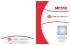### In the box - E3D Online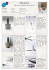### My title - Sociedad Mexicana de Ingeniería Biomédica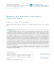### Salginatobel Bridge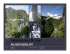### Training Objectives Overview 3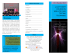### Building Bridges - Bridge of Hope Centre County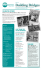### GRUE TOUT-TERRAIN DE 1500 TONNES PRÈS DE LA CHUTE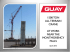### smart pac iii### Pantallas Acusticas Pared Verde (EN)